## ECE1505H Convex Optimization. Lecture 4: Sets and convexity. Taught by Prof. Stark Draper

January 25, 2017 ece1505 No comments , , , , , , ,

ECE1505H Convex Optimization. Lecture 4: Sets and convexity. Taught by Prof. Stark Draper

### Disclaimer

Peeter’s lecture notes from class. These may be incoherent and rough.

These are notes for the UofT course ECE1505H, Convex Optimization, taught by Prof. Stark Draper, covering  content.

### Today

• more on various sets: hyperplanes, half-spaces, polyhedra, balls, ellipses, norm balls, cone of PSD
• generalize inequalities
• operations that preserve convexity
• separating and supporting hyperplanes.

## Hyperplanes

Find some $$\Bx_0 \in \mathbb{R}^n$$ such that $$\Ba^\T \Bx_0 = \Bb$$, so

\begin{equation}\label{eqn:convexOptimizationLecture4:20}
\begin{aligned}
\setlr{ \Bx | \Ba^\T \Bx = \Bb }
&=
\setlr{ \Bx | \Ba^\T \Bx = \Ba^\T \Bx_0 } \\
&=
\setlr{ \Bx | \Ba^\T (\Bx – \Bx_0) } \\
&=
\Bx_0 + \Ba^\perp,
\end{aligned}
\end{equation}

where

\begin{equation}\label{eqn:convexOptimizationLecture4:40}
\Ba^\perp = \setlr{ \Bv | \Ba^\T \Bv = 0 }.
\end{equation}

Recall

\begin{equation}\label{eqn:convexOptimizationLecture4:60}
\Norm{\Bz}_\conj = \sup_\Bx \setlr{ \Bz^\T \Bx | \Norm{\Bx} \le 1 }
\end{equation}

Denote the optimizer of above as $$\Bx^\conj$$. By definition

\begin{equation}\label{eqn:convexOptimizationLecture4:80}
\Bz^\T \Bx^\conj \ge \Bz^\T \Bx \quad \forall \Bx, \Norm{\Bx} \le 1
\end{equation}

This defines a half space in which the unit ball

\begin{equation}\label{eqn:convexOptimizationLecture4:100}
\setlr{ \Bx | \Bz^\T (\Bx – \Bx^\conj \le 0 }
\end{equation}

Start with the $$l_1$$ norm, duals of $$l_1$$ is $$l_\infty$$

Similar pic for $$l_\infty$$, for which the dual is the $$l_1$$ norm, as sketched in fig. 3.  Here the optimizer point is at $$(1,1)$$

and a similar pic for $$l_2$$, which is sketched in fig. 4.

## Polyhedra

\begin{equation}\label{eqn:convexOptimizationLecture4:120}
\begin{aligned}
\mathcal{P}
&= \setlr{ \Bx |
\Ba_j^\T \Bx \le \Bb_j, j \in [1,m],
\Bc_i^\T \Bx = \Bd_i, i \in [1,p]
} \\
&=
\setlr{ \Bx | A \Bx \le \Bb, C \Bx = d },
\end{aligned}
\end{equation}

where the final inequality and equality are component wise.

Proving $$\mathcal{P}$$ is convex:

• Pick $$\Bx_1 \in \mathcal{P}$$, $$\Bx_2 \in \mathcal{P}$$
• Pick any $$\theta \in [0,1]$$
• Test $$\theta \Bx_1 + (1-\theta) \Bx_2$$. Is it in $$\mathcal{P}$$?

\begin{equation}\label{eqn:convexOptimizationLecture4:140}
\begin{aligned}
A \lr{ \theta \Bx_1 + (1-\theta) \Bx_2 }
&=
\theta A \Bx_1 + (1-\theta) A \Bx_2 \\
&\le
\theta \Bb + (1-\theta) \Bb \\
&=
\Bb.
\end{aligned}
\end{equation}

## Balls

Euclidean ball for $$\Bx_c \in \mathbb{R}^n, r \in \mathbb{R}$$

\begin{equation}\label{eqn:convexOptimizationLecture4:160}
\mathcal{B}(\Bx_c, r)
= \setlr{ \Bx | \Norm{\Bx – \Bx_c}_2 \le r },
\end{equation}

or
\begin{equation}\label{eqn:convexOptimizationLecture4:180}
\mathcal{B}(\Bx_c, r)
= \setlr{ \Bx | \lr{\Bx – \Bx_c}^\T \lr{\Bx – \Bx_c} \le r^2 }.
\end{equation}

Let $$\Bx_1, \Bx_2$$, $$\theta \in [0,1]$$

\begin{equation}\label{eqn:convexOptimizationLecture4:200}
\begin{aligned}
\Norm{ \theta \Bx_1 + (1-\theta) \Bx_2 – \Bx_c }_2
&=
\Norm{ \theta (\Bx_1 – \Bx_c) + (1-\theta) (\Bx_2 – \Bx_c) }_2 \\
&\le
\Norm{ \theta (\Bx_1 – \Bx_c)}_2 + \Norm{(1-\theta) (\Bx_2 – \Bx_c) }_2 \\
&=
\Abs{\theta} \Norm{ \Bx_1 – \Bx_c}_2 + \Abs{1 -\theta} \Norm{ \Bx_2 – \Bx_c }_2 \\
&=
\theta \Norm{ \Bx_1 – \Bx_c}_2 + \lr{1 -\theta} \Norm{ \Bx_2 – \Bx_c }_2 \\
&\le
\theta r + (1 – \theta) r \\
&= r
\end{aligned}
\end{equation}

## Ellipse

\begin{equation}\label{eqn:convexOptimizationLecture4:220}
\mathcal{E}(\Bx_c, P)
=
\setlr{ \Bx | (\Bx – \Bx_c)^\T P^{-1} (\Bx – \Bx_c) \le 1 },
\end{equation}

where $$P \in S^n_{++}$$.

• Euclidean ball is an ellipse with $$P = I r^2$$
• Ellipse is image of Euclidean ball $$\mathcal{B}(0,1)$$ under affine mapping.

Given

\begin{equation}\label{eqn:convexOptimizationLecture4:240}
F(\Bu) = P^{1/2} \Bu + \Bx_c
\end{equation}

\begin{equation}\label{eqn:convexOptimizationLecture4:260}
\begin{aligned}
\setlr{ F(\Bu) | \Norm{\Bu}_2 \le r }
&=
\setlr{ P^{1/2} \Bu + \Bx_c | \Bu^\T \Bu \le r^2 } \\
&=
\setlr{ \Bx | \Bx = P^{1/2} \Bu + \Bx_c, \Bu^\T \Bu \le r^2 } \\
&=
\setlr{ \Bx | \Bu = P^{-1/2} (\Bx – \Bx_c), \Bu^\T \Bu \le r^2 } \\
&=
\setlr{ \Bx | (\Bx – \Bx_c)^\T P^{-1} (\Bx – \Bx_c) \le r^2 }
\end{aligned}
\end{equation}

## Geometry of an ellipse

Decomposition of positive definite matrix $$P \in S^n_{++} \subset S^n$$ is:

\begin{equation}\label{eqn:convexOptimizationLecture4:280}
\begin{aligned}
P &= Q \textrm{diag}(\lambda_i) Q^\T \\
Q^\T Q &= 1
\end{aligned},
\end{equation}

where $$\lambda_i \in \mathbb{R}$$, and $$\lambda_i > 0$$. The ellipse is defined by

\begin{equation}\label{eqn:convexOptimizationLecture4:300}
(\Bx – \Bx_c)^\T Q \textrm{diag}(1/\lambda_i) (\Bx – \Bx_c) Q \le r^2
\end{equation}

The term $$(\Bx – \Bx_c)^\T Q$$ projects $$\Bx – \Bx_c$$ onto the columns of $$Q$$. Those columns are perpendicular since $$Q$$ is an orthogonal matrix. Let

\begin{equation}\label{eqn:convexOptimizationLecture4:320}
\tilde{\Bx} = Q^\T (\Bx – \Bx_c),
\end{equation}

this shifts the origin around $$\Bx_c$$ and $$Q$$ rotates into a new coordinate system. The ellipse is therefore

\begin{equation}\label{eqn:convexOptimizationLecture4:340}
\tilde{\Bx}^\T
\begin{bmatrix}
\inv{\lambda_1} & & & \\
&\inv{\lambda_2} & & \\
& \ddots & \\
& & & \inv{\lambda_n}
\end{bmatrix}
\tilde{\Bx}
=
\sum_{i = 1}^n \frac{\tilde{x}_i^2}{\lambda_i} \le 1.
\end{equation}

An example is sketched for $$\lambda_1 > \lambda_2$$ below.

• $$\lambda_i$$ tells us length of the semi-major axis.
• Larger $$\lambda_i$$ means $$\tilde{x}_i^2$$ can be bigger and still satisfy constraint $$\le 1$$.
• Volume of ellipse if proportional to $$\sqrt{ \det P } = \sqrt{ \prod_{i = 1}^n \lambda_i }$$.
• When any $$\lambda_i \rightarrow 0$$ a dimension is lost and the volume goes to zero. That removes the invertibility required.

Ellipses will be seen a lot in this course, since we are interested in “bowl” like geometries (and the ellipse is the image of a Euclidean ball).

## Norm ball.

The norm ball

\begin{equation}\label{eqn:convexOptimizationLecture4:360}
\mathcal{B} = \setlr{ \Bx | \Norm{\Bx} \le 1 },
\end{equation}

is a convex set for all norms. Proof:

Take any $$\Bx, \By \in \mathcal{B}$$

\begin{equation}\label{eqn:convexOptimizationLecture4:380}
\Norm{ \theta \Bx + (1 – \theta) \By }
\le
\Abs{\theta} \Norm{ \Bx } + \Abs{1 – \theta} \Norm{ \By }
=
\theta \Norm{ \Bx } + \lr{1 – \theta} \Norm{ \By }
\lr
\theta + \lr{1 – \theta}
=
1.
\end{equation}

This is true for any p-norm $$1 \le p$$, $$\Norm{\Bx}_p = \lr{ \sum_{i = 1}^n \Abs{x_i}^p }^{1/p}$$.

The shape of a $$p < 1$$ norm unit ball is sketched below (lines connecting points in such a region can exit the region).## Cones

Recall that $$C$$ is a cone if $$\forall \Bx \in C, \theta \ge 0, \theta \Bx \in C$$.

Impt cone of PSD matrices

\begin{equation}\label{eqn:convexOptimizationLecture4:400}
\begin{aligned}
S^n &= \setlr{ X \in \mathbb{R}^{n \times n} | X = X^\T } \\
S^n_{+} &= \setlr{ X \in S^n | \Bv^\T X \Bv \ge 0, \quad \forall v \in \mathbb{R}^n } \\
S^n_{++} &= \setlr{ X \in S^n_{+} | \Bv^\T X \Bv > 0, \quad \forall v \in \mathbb{R}^n } \\
\end{aligned}
\end{equation}

These have respectively

• $$\lambda_i \in \mathbb{R}$$
• $$\lambda_i \in \mathbb{R}_{+}$$
• $$\lambda_i \in \mathbb{R}_{++}$$

$$S^n_{+}$$ is a cone if:

$$X \in S^n_{+}$$, then $$\theta X \in S^n_{+}, \quad \forall \theta \ge 0$$

\begin{equation}\label{eqn:convexOptimizationLecture4:420}
\Bv^\T (\theta X) \Bv
= \theta \Bv^\T \Bv
\ge 0,
\end{equation}

since $$\theta \ge 0$$ and because $$X \in S^n_{+}$$.

Shorthand:

\begin{equation}\label{eqn:convexOptimizationLecture4:440}
\begin{aligned}
X &\in S^n_{+} \Rightarrow X \succeq 0
X &\in S^n_{++} \Rightarrow X \succ 0.
\end{aligned}
\end{equation}

Further $$S^n_{+}$$ is a convex cone.

Let $$A \in S^n_{+}$$, $$B \in S^n_{+}$$, $$\theta_1, \theta_2 \ge 0, \theta_1 + \theta_2 = 1$$, or $$\theta_2 = 1 – \theta_1$$.

Show that $$\theta_1 A + \theta_2 B \in S^n_{+}$$ :

\begin{equation}\label{eqn:convexOptimizationLecture4:460}
\Bv^\T \lr{ \theta_1 A + \theta_2 B } \Bv
=
\theta_1 \Bv^\T A \Bv
+\theta_2 \Bv^\T B \Bv
\ge 0,
\end{equation}

since $$\theta_1 \ge 0, \theta_2 \ge 0, \Bv^\T A \Bv \ge 0, \Bv^\T B \Bv \ge 0$$.

Inequalities:

Start with a proper cone $$K \subseteq \mathbb{R}^n$$

• closed, convex
• non-empty interior (“solid”)
• “pointed” (contains no lines)

The $$K$$ defines a generalized inequality in \R{n} defined as “$$\le_K$$”

Interpreting

\begin{equation}\label{eqn:convexOptimizationLecture4:480}
\begin{aligned}
\Bx \le_K \By &\leftrightarrow \By – \Bx \in K
\Bx \end{aligned}
\end{equation}

Why pointed? Want if $$\Bx \le_K \By$$ and $$\By \le_K \Bx$$ with this $$K$$ is a half space.

Example:1: $$K = \mathbb{R}^n_{+}, \Bx \in \mathbb{R}^n, \By \in \mathbb{R}^n$$

\begin{equation}\label{eqn:convexOptimizationLecture4:500}
\Bx \le_K \By \Rightarrow \By – \Bx \in K
\end{equation}

say:

\begin{equation}\label{eqn:convexOptimizationLecture4:520}
\begin{bmatrix}
y_1 – x_1
y_2 – x_2
\end{bmatrix}
\in R^2_{+}
\end{equation}

Also:

\begin{equation}\label{eqn:convexOptimizationLecture4:540}
K = R^1_{+}
\end{equation}

(pointed, since it contains no rays)

\begin{equation}\label{eqn:convexOptimizationLecture4:560}
\Bx \le_K \By ,
\end{equation}

with respect to $$K = \mathbb{R}^n_{+}$$ means that $$x_i \le y_i$$ for all $$i \in [1,n]$$.

Example:2: For $$K = PSD \subseteq S^n$$,

\begin{equation}\label{eqn:convexOptimizationLecture4:580}
\Bx \le_K \By ,
\end{equation}

means that

\begin{equation}\label{eqn:convexOptimizationLecture4:600}
\By – \Bx \in K = S^n_{+}.
\end{equation}

• Difference $$\By – \Bx$$ is always in $$S$$
• check if in $$K$$ by checking if all eigenvalues $$\ge 0$$.
• $$S^n_{++}$$ is the interior of $$S^n_{+}$$.

Interpretation:

\begin{equation}\label{eqn:convexOptimizationLecture4:620}
\begin{aligned}
\Bx \le_K \By &\leftrightarrow \By – \Bx \in K \\
\Bx \end{aligned}
\end{equation}

We’ll use these with vectors and matrices so often the $$K$$ subscript will often be dropped, writing instead (for vectors)

\begin{equation}\label{eqn:convexOptimizationLecture4:640}
\begin{aligned}
\Bx \le \By &\leftrightarrow \By – \Bx \in \mathbb{R}^n_{+} \\
\Bx < \By &\leftrightarrow \By – \Bx \in \textrm{int} \mathbb{R}^n_{++}
\end{aligned}
\end{equation}

and for matrices

\begin{equation}\label{eqn:convexOptimizationLecture4:660}
\begin{aligned}
\Bx \le \By &\leftrightarrow \By – \Bx \in S^n_{+} \\
\Bx < \By &\leftrightarrow \By – \Bx \in \textrm{int} S^n_{++}.
\end{aligned}
\end{equation}

## Intersection

Take the intersection of (perhaps infinitely many) sets $$S_\alpha$$:

If $$S_\alpha$$ is (affine,convex, conic) for all $$\alpha \in A$$ then

\begin{equation}\label{eqn:convexOptimizationLecture4:680}
\cap_\alpha S_\alpha
\end{equation}

is (affine,convex, conic). To prove in homework:

\begin{equation}\label{eqn:convexOptimizationLecture4:700}
\mathcal{P} = \setlr{ \Bx | \Ba_i^\T \Bx \le \Bb_i, \Bc_j^\T \Bx = \Bd_j, \quad \forall i \cdots j }
\end{equation}

This is convex since the intersection of a bunch of hyperplane and half space constraints.

1. If $$S \subseteq \mathbb{R}^n$$ is convex then\begin{equation}\label{eqn:convexOptimizationLecture4:720}
F(S) = \setlr{ F(\Bx) | \Bx \in S }
\end{equation}is convex.
2. If $$S \subseteq \mathbb{R}^m$$ then\begin{equation}\label{eqn:convexOptimizationLecture4:740}
F^{-1}(S) = \setlr{ \Bx | F(\Bx) \in S }
\end{equation}is convex. Such a mapping is sketched in fig. 14.

# References

 Stephen Boyd and Lieven Vandenberghe. Convex optimization. Cambridge university press, 2004.

## ECE1505H Convex Optimization. Lecture 3: Matrix functions, SVD, and types of Sets. Taught by Prof. Stark Draper

### Disclaimer

Peeter’s lecture notes from class. These may be incoherent and rough.

These are notes for the UofT course ECE1505H, Convex Optimization, taught by Prof. Stark Draper.

## Matrix inner product

Given real matrices $$X, Y \in \mathbb{R}^{m\times n}$$, one possible matrix inner product definition is

\begin{equation}\label{eqn:convexOptimizationLecture3:20}
\begin{aligned}
\innerprod{X}{Y}
&= \textrm{Tr}( X^\T Y) \\
&= \textrm{Tr} \lr{ \sum_{k = 1}^m X_{ki} Y_{kj} } \\
&= \sum_{k = 1}^m \sum_{j = 1}^n X_{kj} Y_{kj} \\
&= \sum_{i = 1}^m \sum_{j = 1}^n X_{ij} Y_{ij}.
\end{aligned}
\end{equation}

This inner product induces a norm on the (matrix) vector space, called the Frobenius norm

\begin{equation}\label{eqn:convexOptimizationLecture3:40}
\begin{aligned}
\Norm{X }_F
&= \textrm{Tr}( X^\T X) \\
&= \sqrt{ \innerprod{X}{X} } \\
&=
\sum_{i = 1}^m \sum_{j = 1}^n X_{ij}^2.
\end{aligned}
\end{equation}

## Range, nullspace.

Definition: Range: Given $$A \in \mathbb{R}^{m \times n}$$, the range of A is the set:

\begin{equation*}
\mathcal{R}(A) = \setlr{ A \Bx | \Bx \in \mathbb{R}^n }.
\end{equation*}

Definition: Nullspace: Given $$A \in \mathbb{R}^{m \times n}$$, the nullspace of A is the set:

\begin{equation*}
\mathcal{N}(A) = \setlr{ \Bx | A \Bx = 0 }.
\end{equation*}

## SVD.

To understand operation of $$A \in \mathbb{R}^{m \times n}$$, a representation of a linear transformation from \R{n} to \R{m}, decompose $$A$$ using the singular value decomposition (SVD).

Definition: SVD: Given $$A \in \mathbb{R}^{m \times n}$$, an operator on $$\Bx \in \mathbb{R}^n$$, a decomposition of the following form is always possible

\begin{equation*}
\begin{aligned}
A &= U \Sigma V^\T \\
U &\in \mathbb{R}^{m \times r} \\
V &\in \mathbb{R}^{n \times r},
\end{aligned}
\end{equation*}

where $$r$$ is the rank of $$A$$, and both $$U$$ and $$V$$ are orthogonal

\begin{equation*}
\begin{aligned}
U^\T U &= I \in \mathbb{R}^{r \times r} \\
V^\T V &= I \in \mathbb{R}^{r \times r}.
\end{aligned}
\end{equation*}

Here $$\Sigma = \textrm{diag}( \sigma_1, \sigma_2, \cdots, \sigma_r )$$, is a diagonal matrix of “singular” values, where

\begin{equation*}
\sigma_1 \ge \sigma_2 \ge \cdots \ge \sigma_r.
\end{equation*}

For simplicity consider square case $$m = n$$

\begin{equation}\label{eqn:convexOptimizationLecture3:100}
A \Bx = \lr{ U \Sigma V^\T } \Bx.
\end{equation}

The first product $$V^\T \Bx$$ is a rotation, which can be checked by looking at the length

\begin{equation}\label{eqn:convexOptimizationLecture3:120}
\begin{aligned}
\Norm{ V^\T \Bx}_2
&= \sqrt{ \Bx^\T V V^\T \Bx } \\
&= \sqrt{ \Bx^\T \Bx } \\
&= \Norm{ \Bx }_2,
\end{aligned}
\end{equation}

which shows that the length of the vector is unchanged after application of the linear transformation represented by $$V^\T$$ so that operation must be a rotation.

Similarly the operation of $$U$$ on $$\Sigma V^\T \Bx$$ also must be a rotation. The operation $$\Sigma = [\sigma_i]_i$$ applies a scaling operation to each component of the vector $$V^\T \Bx$$.

All linear (square) transformations can therefore be thought of as a rotate-scale-rotate operation. Often the $$A$$ of interest will be symmetric $$A = A^\T$$.

## Set of symmetric matrices

Let $$S^n$$ be the set of real, symmetric $$n \times n$$ matrices.

Theorem: Spectral theorem: When $$A \in S^n$$ then it is possible to factor $$A$$ as

\begin{equation*}
A = Q \Lambda Q^\T,
\end{equation*}

where $$Q$$ is an orthogonal matrix, and $$\Lambda = \textrm{diag}( \lambda_1, \lambda_2, \cdots \lambda_n)$$. Here $$\lambda_i \in \mathbb{R} \, \forall i$$ are the (real) eigenvalues of $$A$$.

A real symmetric matrix $$A \in S^n$$ is “positive semi-definite” if

\begin{equation*}
\Bv^\T A \Bv \ge 0 \qquad\forall \Bv \in \mathbb{R}^n, \Bv \ne 0,
\end{equation*}
and is “positive definite” if

\begin{equation*}
\Bv^\T A \Bv > 0 \qquad\forall \Bv \in \mathbb{R}^n, \Bv \ne 0.
\end{equation*}

The set of such matrices is denoted $$S^n_{+}$$, and $$S^n_{++}$$ respectively.

Consider $$A \in S^n_{+}$$ (or $$S^n_{++}$$ )

\begin{equation}\label{eqn:convexOptimizationLecture3:200}
A = Q \Lambda Q^\T,
\end{equation}

possible since the matrix is symmetric. For such a matrix

\begin{equation}\label{eqn:convexOptimizationLecture3:220}
\begin{aligned}
\Bv^\T A \Bv
&=
\Bv^\T Q \Lambda A^\T \Bv \\
&=
\Bw^\T \Lambda \Bw,
\end{aligned}
\end{equation}

where $$\Bw = A^\T \Bv$$. Such a product is

\begin{equation}\label{eqn:convexOptimizationLecture3:240}
\Bv^\T A \Bv
=
\sum_{i = 1}^n \lambda_i w_i^2.
\end{equation}

So, if $$\lambda_i \ge 0$$ ($$\lambda_i > 0$$ ) then $$\sum_{i = 1}^n \lambda_i w_i^2$$ is non-negative (positive) $$\forall \Bw \in \mathbb{R}^n, \Bw \ne 0$$. Since $$\Bw$$ is just a rotated version of $$\Bv$$ this also holds for all $$\Bv$$. A necessary and sufficient condition for $$A \in S^n_{+}$$ ($$S^n_{++}$$ ) is $$\lambda_i \ge 0$$ ($$\lambda_i > 0$$).

## Square root of positive semi-definite matrix

Real symmetric matrix power relationships such as

\begin{equation}\label{eqn:convexOptimizationLecture3:260}
A^2
=
Q \Lambda Q^\T
Q \Lambda Q^\T
=
Q \Lambda^2
Q^\T
,
\end{equation}

or more generally $$A^k = Q \Lambda^k Q^\T,\, k \in \mathbb{Z}$$, can be further generalized to non-integral powers. In particular, the square root (non-unique) of a square matrix can be written

\begin{equation}\label{eqn:convexOptimizationLecture3:280}
A^{1/2} = Q
\begin{bmatrix}
\sqrt{\lambda_1} & & & \\
& \sqrt{\lambda_2} & & \\
& & \ddots & \\
& & & \sqrt{\lambda_n} \\
\end{bmatrix}
Q^\T,
\end{equation}

since $$A^{1/2} A^{1/2} = A$$, regardless of the sign picked for the square roots in question.

## Functions of matrices

Consider $$F : S^n \rightarrow \mathbb{R}$$, and define

\begin{equation}\label{eqn:convexOptimizationLecture3:300}
F(X) = \log \det X,
\end{equation}

Here $$\textrm{dom} F = S^n_{++}$$. The task is to find $$\spacegrad F$$, which can be done by looking at the perturbation $$\log \det ( X + \Delta X )$$

\begin{equation}\label{eqn:convexOptimizationLecture3:320}
\begin{aligned}
\log \det ( X + \Delta X )
&=
\log \det ( X^{1/2} (I + X^{-1/2} \Delta X X^{-1/2}) X^{1/2} ) \\
&=
\log \det ( X (I + X^{-1/2} \Delta X X^{-1/2}) ) \\
&=
\log \det X + \log \det (I + X^{-1/2} \Delta X X^{-1/2}).
\end{aligned}
\end{equation}

Let $$X^{-1/2} \Delta X X^{-1/2} = M$$ where $$\lambda_i$$ are the eigenvalues of $$M : M \Bv = \lambda_i \Bv$$ when $$\Bv$$ is an eigenvector of $$M$$. In particular

\begin{equation}\label{eqn:convexOptimizationLecture3:340}
(I + M) \Bv =
(1 + \lambda_i) \Bv,
\end{equation}

where $$1 + \lambda_i$$ are the eigenvalues of the $$I + M$$ matrix. Since the determinant is the product of the eigenvalues, this gives

\begin{equation}\label{eqn:convexOptimizationLecture3:360}
\begin{aligned}
\log \det ( X + \Delta X )
&=
\log \det X +
\log \prod_{i = 1}^n (1 + \lambda_i) \\
&=
\log \det X +
\sum_{i = 1}^n \log (1 + \lambda_i).
\end{aligned}
\end{equation}

If $$\lambda_i$$ are sufficiently “small”, then $$\log ( 1 + \lambda_i ) \approx \lambda_i$$, giving

\begin{equation}\label{eqn:convexOptimizationLecture3:380}
\log \det ( X + \Delta X )
=
\log \det X +
\sum_{i = 1}^n \lambda_i
\approx
\log \det X +
\textrm{Tr}( X^{-1/2} \Delta X X^{-1/2} ).
\end{equation}

Since
\begin{equation}\label{eqn:convexOptimizationLecture3:400}
\textrm{Tr}( A B ) = \textrm{Tr}( B A ),
\end{equation}

this trace operation can be written as

\begin{equation}\label{eqn:convexOptimizationLecture3:420}
\log \det ( X + \Delta X )
\approx
\log \det X +
\textrm{Tr}( X^{-1} \Delta X )
=
\log \det X +
\innerprod{ X^{-1}}{\Delta X},
\end{equation}

so
\begin{equation}\label{eqn:convexOptimizationLecture3:440}
\end{equation}

To check this, consider the simplest example with $$X \in \mathbb{R}^{1 \times 1}$$, where we have

\begin{equation}\label{eqn:convexOptimizationLecture3:460}
\frac{d}{dX} \lr{ \log \det X } = \frac{d}{dX} \lr{ \log X } = \inv{X} = X^{-1}.
\end{equation}

This is a nice example demonstrating how the gradient can be obtained by performing a first order perturbation of the function. The gradient can then be read off from the result.

## Second order perturbations

• To get first order approximation found the part that varied linearly in $$\Delta X$$.
• To get the second order part, perturb $$X^{-1}$$ by $$\Delta X$$ and see how that perturbation varies in $$\Delta X$$.

For $$G(X) = X^{-1}$$, this is

\begin{equation}\label{eqn:convexOptimizationLecture3:480}
\begin{aligned}
(X + \Delta X)^{-1}
&=
\lr{ X^{1/2} (I + X^{-1/2} \Delta X X^{-1/2} ) X^{1/2} }^{-1} \\
&=
X^{-1/2} (I + X^{-1/2} \Delta X X^{-1/2} )^{-1} X^{-1/2}
\end{aligned}
\end{equation}

To be proven in the homework (for “small” A)

\begin{equation}\label{eqn:convexOptimizationLecture3:500}
(I + A)^{-1} \approx I – A.
\end{equation}

This gives

\begin{equation}\label{eqn:convexOptimizationLecture3:520}
\begin{aligned}
(X + \Delta X)^{-1}
&=
X^{-1/2} (I – X^{-1/2} \Delta X X^{-1/2} ) X^{-1/2} \\
&=
X^{-1} – X^{-1} \Delta X X^{-1},
\end{aligned}
\end{equation}

or

\begin{equation}\label{eqn:convexOptimizationLecture3:800}
\begin{aligned}
G(X + \Delta X)
&= G(X) + (D G) \Delta X \\
&= G(X) + (\spacegrad G)^\T \Delta X,
\end{aligned}
\end{equation}

so
\begin{equation}\label{eqn:convexOptimizationLecture3:820}
=
– X^{-1} \Delta X X^{-1}.
\end{equation}

The Taylor expansion of $$F$$ to second order is

\begin{equation}\label{eqn:convexOptimizationLecture3:840}
F(X + \Delta X)
=
F(X)
+
\textrm{Tr} \lr{ (\spacegrad F)^\T \Delta X}
+
\inv{2}
\lr{ (\Delta X)^\T (\spacegrad^2 F) \Delta X}.
\end{equation}

The first trace can be expressed as an inner product

\begin{equation}\label{eqn:convexOptimizationLecture3:860}
\begin{aligned}
\textrm{Tr} \lr{ (\spacegrad F)^\T \Delta X}
&=
\innerprod{ \spacegrad F }{\Delta X} \\
&=
\innerprod{ X^{-1} }{\Delta X}.
\end{aligned}
\end{equation}

The second trace also has the structure of an inner product

\begin{equation}\label{eqn:convexOptimizationLecture3:880}
\begin{aligned}
(\Delta X)^\T (\spacegrad^2 F) \Delta X
&=
\textrm{Tr} \lr{ (\Delta X)^\T (\spacegrad^2 F) \Delta X} \\
&=
\innerprod{ (\spacegrad^2 F)^\T \Delta X }{\Delta X},
\end{aligned}
\end{equation}

where a no-op trace could be inserted in the second order term since that quadratic form is already a scalar. This $$(\spacegrad^2 F)^\T \Delta X$$ term has essentially been found implicitly by performing the linear variation of $$\spacegrad F$$ in $$\Delta X$$, showing that we must have

\begin{equation}\label{eqn:convexOptimizationLecture3:900}
\textrm{Tr} \lr{ (\Delta X)^\T (\spacegrad^2 F) \Delta X}
=
\innerprod{ – X^{-1} \Delta X X^{-1} }{\Delta X},
\end{equation}

so
\begin{equation}\label{eqn:convexOptimizationLecture3:560}
F( X + \Delta X) = F(X) +
\innerprod{X^{-1}}{\Delta X}
+\inv{2} \innerprod{-X^{-1} \Delta X X^{-1}}{\Delta X},
\end{equation}

or
\begin{equation}\label{eqn:convexOptimizationLecture3:580}
\log \det ( X + \Delta X) = \log \det X +
\textrm{Tr}( X^{-1} \Delta X )
– \inv{2} \textrm{Tr}( X^{-1} \Delta X X^{-1} \Delta X ).
\end{equation}

## Convex Sets

• Types of sets: Affine, convex, cones
• Examples: Hyperplanes, polyhedra, balls, ellipses, norm balls, cone of PSD matrices.

Definition: Affine set:

A set $$C \subseteq \mathbb{R}^n$$ is affine if $$\forall \Bx_1, \Bx_2 \in C$$ then

\begin{equation*}
\theta \Bx_1 + (1 -\theta) \Bx_2 \in C, \qquad \forall \theta \in \mathbb{R}.
\end{equation*}

The affine sum above can
be rewritten as

\begin{equation}\label{eqn:convexOptimizationLecture3:600}
\Bx_2 + \theta (\Bx_1 – \Bx_2).
\end{equation}

Since $$\theta$$ is a scaling, this is the line containing $$\Bx_2$$ in the direction between $$\Bx_1$$ and $$\Bx_2$$.

Observe that the solution to a set of linear equations

\begin{equation}\label{eqn:convexOptimizationLecture3:620}
C = \setlr{ \Bx | A \Bx = \Bb },
\end{equation}

is an affine set. To check, note that

\begin{equation}\label{eqn:convexOptimizationLecture3:640}
\begin{aligned}
A (\theta \Bx_1 + (1 – \theta) \Bx_2)
&=
\theta A \Bx_1 + (1 – \theta) A \Bx_2 \\
&=
\theta \Bb + (1 – \theta) \Bb \\
&= \Bb.
\end{aligned}
\end{equation}

Definition: Affine combination: An affine combination of points $$\Bx_1, \Bx_2, \cdots \Bx_n$$ is

\begin{equation*}
\sum_{i = 1}^n \theta_i \Bx_i,
\end{equation*}

such that for $$\theta_i \in \mathbb{R}$$

\begin{equation*}
\sum_{i = 1}^n \theta_i = 1.
\end{equation*}

An affine set contains all affine combinations of points in the set. Examples of a couple affine sets are sketched in fig 1.1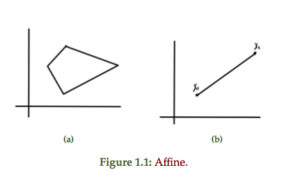For comparison, a couple of non-affine sets are sketched in fig 1.2Definition: Convex set: A set $$C \subseteq \mathbb{R}^n$$ is convex if $$\forall \Bx_1, \Bx_2 \in C$$ and $$\forall \theta \in \mathbb{R}, \theta \in [0,1]$$, the combination

\begin{equation}\label{eqn:convexOptimizationLecture3:700}
\theta \Bx_1 + (1 – \theta) \Bx_2 \in C.
\end{equation}

Definition: Convex combination: A convex combination of $$\Bx_1, \Bx_2, \cdots \Bx_n$$ is

\begin{equation*}
\sum_{i = 1}^n \theta_i \Bx_i,
\end{equation*}

such that $$\forall \theta_i \ge 0$$

\begin{equation*}
\sum_{i = 1}^n \theta_i = 1
\end{equation*}

Definition: Convex hull: Convex hull of a set $$C$$ is a set of all convex combinations of points in $$C$$, denoted

\begin{equation}\label{eqn:convexOptimizationLecture3:720}
\textrm{conv}(C) = \setlr{ \sum_{i=1}^n \theta_i \Bx_i | \Bx_i \in C, \theta_i \ge 0, \sum_{i=1}^n \theta_i = 1 }.
\end{equation}

A non-convex set can be converted into a convex hull by filling in all the combinations of points connecting points in the set, as sketched in fig 1.3.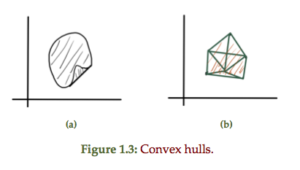Definition: Cone: A set $$C$$ is a cone if $$\forall \Bx \in C$$ and $$\forall \theta \ge 0$$ we have $$\theta \Bx \in C$$.

This scales out if $$\theta > 1$$ and scales in if $$\theta < 1$$.

A convex cone is a cone that is also a convex set. A conic combination is

\begin{equation*}
\sum_{i=1}^n \theta_i \Bx_i, \theta_i \ge 0.
\end{equation*}

A convex and non-convex 2D cone is sketched in fig. 1.4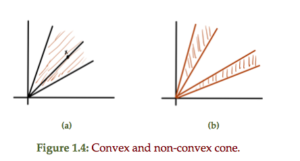A comparison of properties for different set types is tabulated in table 1.1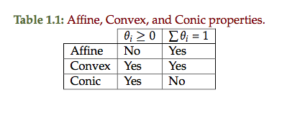## Hyperplanes and half spaces

Definition: Hyperplane: A hyperplane is defined by

\begin{equation*}
\setlr{ \Bx | \Ba^\T \Bx = \Bb, \Ba \ne 0 }.
\end{equation*}

A line and plane are examples of this general construct as sketched in
fig. 1.5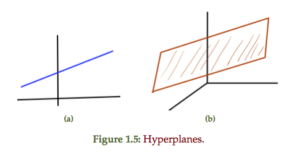An alternate view is possible should one
find any specific $$\Bx_0$$ such that $$\Ba^\T \Bx_0 = \Bb$$

\begin{equation}\label{eqn:convexOptimizationLecture3:740}
\setlr{\Bx | \Ba^\T \Bx = b }
=
\setlr{\Bx | \Ba^\T (\Bx -\Bx_0) = 0 }
\end{equation}

This shows that $$\Bx – \Bx_0 = \Ba^\perp$$ is perpendicular to $$\Ba$$, or

\begin{equation}\label{eqn:convexOptimizationLecture3:780}
\Bx
=
\Bx_0 + \Ba^\perp.
\end{equation}

This is the subspace perpendicular to $$\Ba$$ shifted by $$\Bx_0$$, subject to $$\Ba^\T \Bx_0 = \Bb$$. As a set

\begin{equation}\label{eqn:convexOptimizationLecture3:760}
\Ba^\perp = \setlr{ \Bv | \Ba^\T \Bv = 0 }.
\end{equation}

## Half space

Definition: Half space: The half space is defined as
\begin{equation*}
\setlr{ \Bx | \Ba^\T \Bx = \Bb }
= \setlr{ \Bx | \Ba^\T (\Bx – \Bx_0) \le 0 }.
\end{equation*}

This can also be expressed as $$\setlr{ \Bx | \innerprod{ \Ba }{\Bx – \Bx_0 } \le 0 }$$.